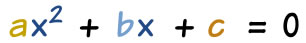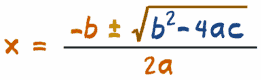# 二次方程式解算器

## 是不是 二次？x2 = 3x −1 x2 − 3x + 1 = 0 a=1, b=−3, c=1
2(x2 − 2x) = 5 2x2 − 4x − 5 = 0 a=2, b=−4, c=−5
x(x−1) = 3 x2 − x − 3 = 0 a=1, b=−1, c=−3
5 + 1/x − 1/x2 = 0 5x2 + x − 1 = 0 a=5, b=1, c=−1

## 怎样解算？"±" 的意思是你要加和减，故此方程式通常有两个答案!